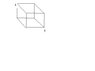# Electromag Potential difference

lektor
Hello forum dwellers :D

I recent have been coming across questions which deal with large arrangements of resistors and in other cases circuits with open switches where the goal is to calculate the potential difference.

These questions have been causing me quite a bit of strife and i was hoping for some help on a few of worse ones.

1) Shown is a wire cube made from wire that has a resistance of R along each of its twelve sides. What is the resistance of the network of resistors between points A and B?

#### Attachments

•resistors.JPG
3.2 KB · Views: 291

lektor
And next, one of these potential difference questions I have talked about

Homework Helper
1) The diagram is not opening still I can guess that the AB will be a body diagonal, a face diagonal or a side. In any of the case knowing the equipotential points, and considering them connected through conducting wires will not change the currents but the circuit becomes quiet simple. Have a try. Best of luck!

lektor
Hey, i noticed you used some terms.. "a body diagonal, a face diagonal or a side" i am not aware of what these mean.

And also the aim is to find the resistance between points, I am not sure if you were looking for that or the voltage in your post.. ?

cheers

Homework Helper
yas

Now I can see your first diagram. AB is the body diagonal. Each surface of a cube is a square and the diagonal of any square surface of the cube is called face diagonal. I think you know the side of a cube.

Homework Helper
For the first problem from A the three sides (resistors) A1, A2 and A3 are symmetrical with point A. Similarly these points are symmetrical about point B. Hence with our commonsense we can guess that the three pains are equipotential and so the three resistances A1, A2 and A3 may be considered parallel.

Similarly points 4, 5, and 6 are equipotential. So resistances 4B, 5B and 6B are in parallel.

Rest of six are connected such that one end is with 1, 2 or 3 and the other point is with 4,5, of 6 and so parallel with each other.

In total we can say that the first three resistance are in parallel then in series with next six,which are in parallel, and then in series with next three which are parallel.
gives total resistance 5R/6.

#### Attachments

• cube.doc
19 KB · Views: 132
Last edited: